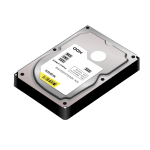# How many megabytes in a gigabyte?Question: How many megabytes in a gigabyte?

Answer: There are 1000 megabyte in 1 gigabyte.

1000 megabyte (1 MB) = 1 gigabyte
1000 gigabyte (1 GB) = 1 terabyte
1000 terabyte (1 TB) = 1 petabyte
1000 petabyte (1 PB) = 1 exabyte

When it comes to data transfer or disk space. Most web hosts usually use the decimal system (above examples) and not the binary system (used by RAM/computer memory manufacturers). If you want to figure out what you will be receiving exactly from your web host then you should inquire with your web host.

1 megabit (1 Mbit) = 1000 kilobits
1 megabyte (1 MB) = 1000 kilobytes
1 mebibyte (1 MiB) = 1024 kibibytes

1 gigabit (1 Gbit) = 1000 megabits
1 gigabyte (1 GB) = 1000 megabytes
1 gibibyte (1 GiB) = 1024 mebibytes

1 terabit (1 Tbit) = 1000 gigabits
1 terabyte (1 TB) = 1000 gigabytes
1 tebibyte (1 TiB) = 1024 gibibytes